# Setup for 4 Player a Game of Food Chain Magnate

Originally generated on 12/1/2018 2:45:53 PM
A direct link to this setup is https://www.BoardgameHelpers.com/FoodChainMagnate/LoadGame.aspx?id=\$2a\$04\$QYoq56c6kbTyN4Gsz9q2WO

## Player Setup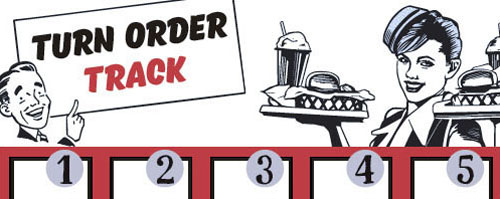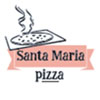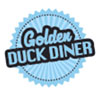## Other Setup

Bank starts with \$200.00
Remove these Billboards: #16
Number of 1x Employee cards used = 2

## Map (Random)

Click here to view Map Tile Lettering Key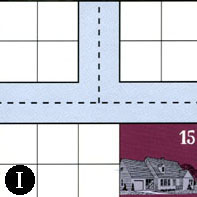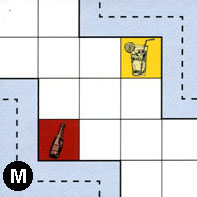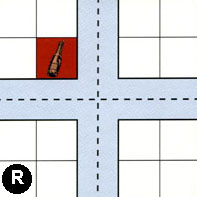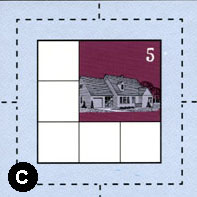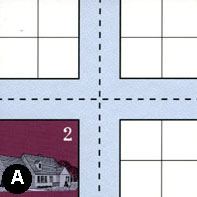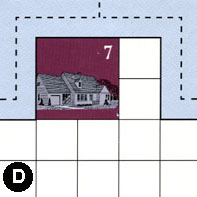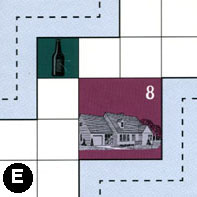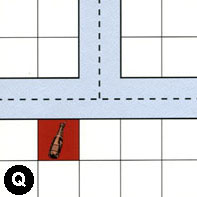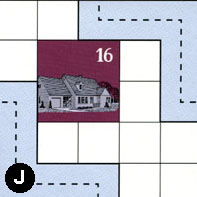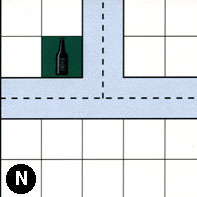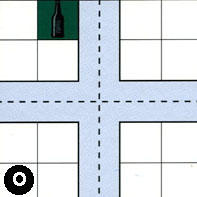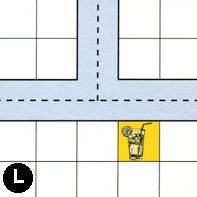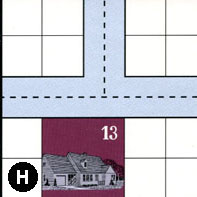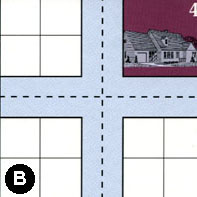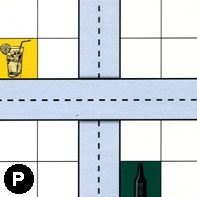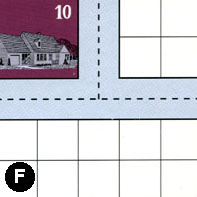### Map Stats

Map Option = Random
Total Number of Tiles = 16
Total Number of Starting Houses = 9
Total Number of Beer Spots = 4
Total Number of Soda Spots = 3
Total Number of Lemonade Spots = 3
Total Number of Drink Spots = 10
Number of independent path systems = 3
Number of independent neighborhoods = 15

Path System 1
Size = Medium
Number of Tiles = 11
Number of Paths = 32
Contains Loop(s) = No
Number of Starting Houses = 7
Starting Houses Pct of Map (%) = 78
Number of Beer Spots = 4
Number of Soda Spots = 1
Number of Lemonade Spots = 1
Number of Drink Spots = 6
Drink Spots Pct of Map (%) = 60
Tile Ids = B, D, E, F, H, I, J, M, N, O, P
Paths = IWN, IWE, MWS, DWN, EWN, OWN, OWE, ONE, ONS, PNS, OWS, OES, DNE, NWE, BWS, PWE, FWE, FWN, FNE, BWN, BNE, HWN, JEN, NWN, BNS, BES, HNE, HWE, BWE, NNE, DWE, INE What do these mean?
Starting House Ids = 0, 4, 7, 10, 13, 15, 16
Path System 2
Size = Medium
Number of Tiles = 6
Number of Paths = 20
Contains Loop(s) = No
Number of Starting Houses = 3
Starting Houses Pct of Map (%) = 33
Number of Beer Spots = 0
Number of Soda Spots = 2
Number of Lemonade Spots = 2
Number of Drink Spots = 4
Drink Spots Pct of Map (%) = 40
Tile Ids = C, E, L, M, Q, R
Paths = MEN, RWE, CWN, QWE, LWN, LNE, LWE, QWN, EES, RWN, CNE, CWE, QNE, CWS, CNS, RWS, RNS, RNE, RES, CES What do these mean?
Starting House Ids = 0, 5, 8
Path System 3
Size = Medium
Number of Tiles = 2
Number of Paths = 7
Contains Loop(s) = No
Number of Starting Houses = 2
Starting Houses Pct of Map (%) = 22
Number of Beer Spots = 0
Number of Soda Spots = 0
Number of Lemonade Spots = 0
Number of Drink Spots = 0
Drink Spots Pct of Map (%) = 0
Tile Ids = A, J
Paths = AWS, AWN, ANE, JWS, AWE, AES, ANS What do these mean?
Starting House Ids = 2, 16

Neighborhood 1
Total Size = Small
Number of Total Spaces = 4
Number of Empty Spaces (for new houses & gardens) = 4
Number of Beer Spots = 0
Number of Soda Spots = 0
Number of Lemonade Spots = 0
Number of Drink Spots = 0
Number of Starting Houses = 0
Starting Houses Pct of Map (%) = 0
Tile Ids = I
Neighborhood 2
Total Size = Large
Number of Total Spaces = 78
Number of Empty Spaces (for new houses & gardens) = 70
Number of Beer Spots = 1
Number of Soda Spots = 1
Number of Lemonade Spots = 2
Number of Drink Spots = 4
Number of Starting Houses = 1
Starting Houses Pct of Map (%) = 11
Tile Ids = E, F, I, L, M, O, P, Q, R
Starting House Ids = 8
Neighborhood 3
Total Size = Small
Number of Total Spaces = 4
Number of Empty Spaces (for new houses & gardens) = 4
Number of Beer Spots = 0
Number of Soda Spots = 0
Number of Lemonade Spots = 0
Number of Drink Spots = 0
Number of Starting Houses = 0
Starting Houses Pct of Map (%) = 0
Tile Ids = R
Neighborhood 4
Total Size = Small
Number of Total Spaces = 4
Number of Empty Spaces (for new houses & gardens) = 4
Number of Beer Spots = 0
Number of Soda Spots = 0
Number of Lemonade Spots = 0
Number of Drink Spots = 0
Number of Starting Houses = 0
Starting Houses Pct of Map (%) = 0
Tile Ids = R
Neighborhood 5
Total Size = Medium
Number of Total Spaces = 9
Number of Empty Spaces (for new houses & gardens) = 5
Number of Beer Spots = 0
Number of Soda Spots = 0
Number of Lemonade Spots = 0
Number of Drink Spots = 0
Number of Starting Houses = 1
Starting Houses Pct of Map (%) = 11
Tile Ids = C
Starting House Ids = 5
Neighborhood 6
Total Size = Large
Number of Total Spaces = 67
Number of Empty Spaces (for new houses & gardens) = 47
Number of Beer Spots = 0
Number of Soda Spots = 0
Number of Lemonade Spots = 0
Number of Drink Spots = 0
Number of Starting Houses = 5
Starting Houses Pct of Map (%) = 56
Tile Ids = A, D, H, I, J, N
Starting House Ids = 2, 7, 13, 15, 16
Neighborhood 7
Total Size = Medium
Number of Total Spaces = 8
Number of Empty Spaces (for new houses & gardens) = 7
Number of Beer Spots = 0
Number of Soda Spots = 1
Number of Lemonade Spots = 0
Number of Drink Spots = 1
Number of Starting Houses = 0
Starting Houses Pct of Map (%) = 0
Tile Ids = Q, R
Neighborhood 8
Total Size = Medium
Number of Total Spaces = 14
Number of Empty Spaces (for new houses & gardens) = 13
Number of Beer Spots = 0
Number of Soda Spots = 1
Number of Lemonade Spots = 0
Number of Drink Spots = 1
Number of Starting Houses = 0
Starting Houses Pct of Map (%) = 0
Tile Ids = L, Q
Neighborhood 9
Total Size = Small
Number of Total Spaces = 4
Number of Empty Spaces (for new houses & gardens) = 4
Number of Beer Spots = 0
Number of Soda Spots = 0
Number of Lemonade Spots = 0
Number of Drink Spots = 0
Number of Starting Houses = 0
Starting Houses Pct of Map (%) = 0
Tile Ids = A
Neighborhood 10
Total Size = Medium
Number of Total Spaces = 26
Number of Empty Spaces (for new houses & gardens) = 24
Number of Beer Spots = 1
Number of Soda Spots = 0
Number of Lemonade Spots = 1
Number of Drink Spots = 2
Number of Starting Houses = 0
Starting Houses Pct of Map (%) = 0
Tile Ids = B, N, O, P
Neighborhood 11
Total Size = Medium
Number of Total Spaces = 12
Number of Empty Spaces (for new houses & gardens) = 11
Number of Beer Spots = 1
Number of Soda Spots = 0
Number of Lemonade Spots = 0
Number of Drink Spots = 1
Number of Starting Houses = 0
Starting Houses Pct of Map (%) = 0
Tile Ids = B, H, N
Neighborhood 12
Total Size = Medium
Number of Total Spaces = 8
Number of Empty Spaces (for new houses & gardens) = 4
Number of Beer Spots = 0
Number of Soda Spots = 0
Number of Lemonade Spots = 0
Number of Drink Spots = 0
Number of Starting Houses = 1
Starting Houses Pct of Map (%) = 11
Tile Ids = B, H
Starting House Ids = 4
Neighborhood 13
Total Size = Medium
Number of Total Spaces = 8
Number of Empty Spaces (for new houses & gardens) = 8
Number of Beer Spots = 0
Number of Soda Spots = 0
Number of Lemonade Spots = 0
Number of Drink Spots = 0
Number of Starting Houses = 0
Starting Houses Pct of Map (%) = 0
Tile Ids = B, P
Neighborhood 14
Total Size = Medium
Number of Total Spaces = 8
Number of Empty Spaces (for new houses & gardens) = 7
Number of Beer Spots = 1
Number of Soda Spots = 0
Number of Lemonade Spots = 0
Number of Drink Spots = 1
Number of Starting Houses = 0
Starting Houses Pct of Map (%) = 0
Tile Ids = F, P
Neighborhood 15
Total Size = Small
Number of Total Spaces = 4
Number of Empty Spaces (for new houses & gardens) = 0
Number of Beer Spots = 0
Number of Soda Spots = 0
Number of Lemonade Spots = 0
Number of Drink Spots = 0
Number of Starting Houses = 1
Starting Houses Pct of Map (%) = 11
Tile Ids = F
Starting House Ids = 10

v2.1.5